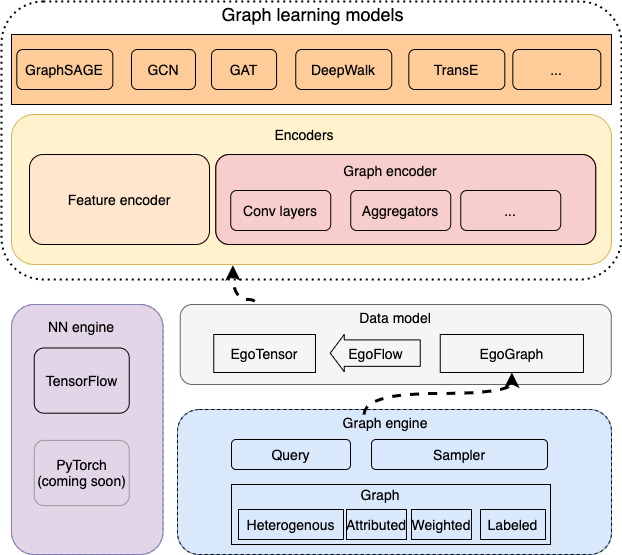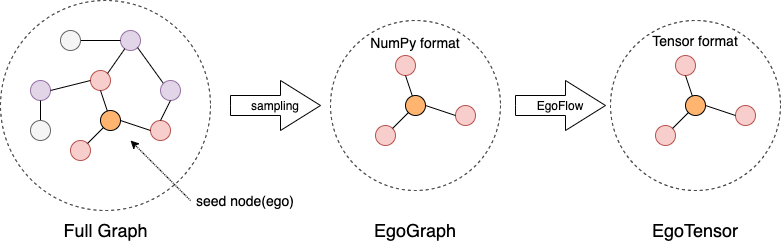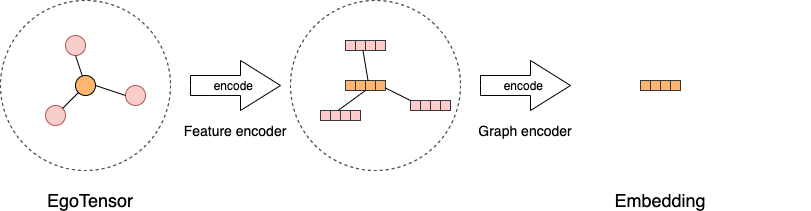# GraphScope Learning Engine¶

The learning engine in GraphScope (GLE) drives from Graph-Learn, a distributed framework designed for development and training of large-scale graph neural networks (GNNs). GLE provides a programming interface carefully designed for the development of graph neural network models, and has been widely applied in many scenarios within Alibaba, such as search recommendation, network security, and knowledge graphs.

Next, we will walk through a quick-start turtorial on how to build a user-defined GNN model using GLE.

## Graph Learning Model¶

There are two ways to train a graph learning model. One is to compute based on the whole graph directly. The GCN and GAT are originally proposed using this approach, directly computing on the entire adjacency matrix. However, this approach will consume huge amount of memory on large-scale graphs, limiting its applicability. The other approach is to sample subgraphs from the whole graph, and use batch training that is commonly used in deep learning. The typical examples include GraphSAGE，FastGCN and GraphSAINT methods.

GLE is designed for large-scale graph neural networks. It consists of an efficient graph engine, a set of user-friendly APIs, and a rich set of built-in popular GNN models. The graph engine stores the graph topology and attributes distributedly, and support efficient graph sampling amd query. It can work with popular tensor engines including TensorFlow and PyTorch. In the following, our model implemnetations are based on TensorFlow.### Data model¶

To build and train a model, GLE usually samples subgraphs as the training data, and perform batch training with it. We start with introducing the basic data model.

`EgoGraph` is the underlying data model in GLE. It consists of a batch of seed nodes or edges(named ‘ego’) and their receptive fields (multi-hops neighbors). We implement many build-in samplers to traverse the graph and sample the neighbors. Negative samplers are also implemented for unsupervised training.

The sampled data grouped in `EgoGraph` is organized into numpy format. It can be converted to different tensor formats, `EgoTensor`, based on the different deep learning engine. GLE uses `EgoFlow` to convert `EgoGraph` to `EgoTensor`. And the `EgoTensor` serves as the training data.### Encoder¶

A graph learning model can be viewed as using an encoder to encode the `EgoTensor` of a node, edge or subgraph into a vector.

GLE first uses feature encoders to encode raw features of nodes or edges, and the produced feature embeddings are then encoded by different graph encoders to produce the final embedding vectors. For most of GNN models, graph encoders provide a way to generate an abstraction of a target node or edge by aggregating information from its neighbors. This aggregation and encoding are usually implemented by many different graph convolutional layers.Based on the data models and encoders, one can easily implement different graph learning models. We introduce in detail how to develope a GNN model in the next section.

In this document, we will introduce how to use the basic APIs provided by GLE to cooperate with deep learning engines, such as TensorFlow, to build graph learning algorithms. We demonstrate the GCN model as an example which is one of the most popular models in graph neural network.

In general, it requires the following four steps to build an algorithm.

• Specify sampling mode: use graph sampling and query methods to sample subgraphs and organize them into `EgoGraph`

We abstract out four basic functions, `sample_seed`, `positive_sample`, `negative_sample` and `receptive_fn`. To generate `Node` or `Edges`, we use `sample_seed` to traverse the graph. Then, we use `positive_sample` with `Nodes` or `Edges` as inputs to generate positive samples for training. For unsupervised learning `negative_sample` produces negative samples. GNNs need to aggregate neighbor information so that we abstract `receptive_fn` to sample neighbors. Finally, the `Nodes` and `Edges` produced by `sample_seed`, and their sampled neighbors form an `EgoGraph`.

• Construct graph data flow: convert `EgoGraph` to `EgoTensor` using `EgoFlow`

GLE algorithm model is based on a deep learning engine similar to TensorFlow. As a result, it requires to convert the sampled `EgoGraph`s to the tensor format `EgoTensor`, which is encapsulated in `EgoFlow` that can generate an iterator for batch training.

• Define encoder: Use `EgoGraph` encoder and feature encoder to encode `EgoTensor`

After getting the `EgoTensor`, we first encode the original nodes and edge features into vectors using common feature encoders. Then, we feed the vectors into a GNN model as the feature input. Next, we use the graph encoder to process the `EgoTensor`, combining the neighbor node features with its characteristics to get the nodes or edge vectors.

• Design loss functions and training processes: select the appropriate loss function and write the training process.

GLE has built-in common loss functions and optimizers. It also encapsulates the training process. GLE supports both single-machine and distributed training. Users can also customize the loss functions, optimizers and training processes.

Next, we introduce how to implement a GCN model using the above four steps.

### Sampling¶

We use the Cora dataset as the node classification example. We provide a simple data conversion script `cora.py` to convert the original Cora to the format required by GLE. The script generates following 5 files: node_table, edge_table_with_self_loop, train_table, val_table and test_table. They are the node table, the edge table, and the nodes tables used to distinguish training, validation, and testing sets.

Then, we can construct the graph using the following code snippet.

```import graphlearn as gle
g = gle.Graph()\
.node(dataset_folder + "node_table", node_type=node_type,
decoder=gle.Decoder(labeled=True,
attr_types=["float"] * 1433,
attr_delimiter=":"))\
.edge(dataset_folder + "edge_table_with_self_loop",
edge_type=(node_type, node_type, edge_type),
decoder=gle.Decoder(weighted=True), directed=False)\
.node(dataset_folder + "train_table", node_type="train",
decoder=gle.Decoder(weighted=True))\
.node(dataset_folder + "val_table", node_type="val",
decoder=gle.Decoder(weighted=True))\
.node(dataset_folder + "test_table", node_type="test",
decoder=gle.Decoder(weighted=True))
```

We load the graph into memory by calling `g.init()`.

```class GCN(gle.LearningBasedModel):
def __init__(self,
graph,
output_dim,
features_num,
batch_size,
categorical_attrs_desc='',
hidden_dim=16,
hops_num=2,):
self.graph = graph
self.batch_size = batch_size
```

The GCN model inherits from the basic learning model class `LearningBasedModel`. As a result, we only need to override the sampling, model construction, and other methods to build GCN model.

```class GCN(gle.LearningBasedModel):
# ...
def _sample_seed(self):
return self.graph.V('train').batch(self.batch_size).values()

def _positive_sample(self, t):
return gle.Edges(t.ids, self.node_type,
t.ids, self.node_type,
self.edge_type, graph=self.graph)

def _receptive_fn(self, nodes):
return self.graph.V(nodes.type, feed=nodes).alias('v') \
.outV(self.edge_type).sample().by('full').alias('v1') \
.outV(self.edge_type).sample().by('full').alias('v2') \
.emit(lambda x: gle.EgoGraph(x['v'], [ag.Layer(nodes=x['v1']), ag.Layer(nodes=x['v2'])]))
```

`_sample_seed` and `_positive_sample` use to sample seed nodes and positive samples. `_receptive_fn` samples neighbors and organizes `EgoGraph`. `OutV` returns one-hop neighbors so the above code samples two-hop neighbors. Users can choose different neighbor sampling methods. For the original GCN, it requires all neighbors of each node are so we use ‘full’ for sampling. We aggregate the sampling results in `EgoGraph` which is the return value.

### Graph Data Flow¶

In `build` function, we convert `EgoGraph` to `EgoTensor` using `EgoFlow`. `EgoFlow` contains an data flow iterator and several `EgoTensor`s.

```class GCN(gle.LearningBasedModel):
def build(self):
ego_flow = gle.EgoFlow(self._sample_seed,
self._positive_sample,
self._receptive_fn,
self.src_ego_spec)
iterator = ego_flow.iterator
pos_src_ego_tensor = ego_flow.pos_src_ego_tensor
# ...
```

We can get the `EgoTensor` corresponding to the previous `EgoGraph` from `EgoFlow`.

### Model¶

Next, we first use the feature encoder to encode the original features. In this example, we use `IdentityEncoder` that returns itself, because the features of Cora are already in vector formats. For both the discrete and continuous features, we can use `WideNDeepEncoder`, To learn more encoders, please refer to feature encoder. Then, we use the `GCNConv` layer to construct the graph encoder. For each node in GCN, we sample all of its neighbors, and organize them in a sparse format. Therefore, we use `SparseEgoGraphEncoder`. For the neighbor-aligned model, please refer to the implementation of GraphSAGE.

```class GCN(gle.LearningBasedModel):
def _encoders(self):
depth = self.hops_num
feature_encoders = [gle.encoders.IdentityEncoder()] * (depth + 1)
conv_layers = []
# for input layer
conv_layers.append(gle.layers.GCNConv(self.hidden_dim))
# for hidden layer
for i in range(1, depth - 1):
conv_layers.append(gle.layers.GCNConv(self.hidden_dim))
# for output layer
conv_layers.append(gle.layers.GCNConv(self.output_dim, act=None))
encoder = gle.encoders.SparseEgoGraphEncoder(feature_encoders,
conv_layers)
return {"src": encoder, "edge": None, "dst": None}
```

### Loss Function and Training Process¶

For the Cora node classification model, we can select the corresponding classification loss function in TensorFlow. Then, we combine the encoder and loss function in the `build` function, and finally return a data iterator and a loss function.

```class GCN(gle.LearningBasedModel):
# ...
def _supervised_loss(self, emb, label):
return tf.reduce_mean(tf.nn.sparse_softmax_cross_entropy_with_logits(emb, label))

def build(self):
ego_flow = gle.EgoFlow(self._sample_seed,
self._positive_sample,
self._receptive_fn,
self.src_ego_spec,
full_graph_mode=self.full_graph_mode)
iterator = ego_flow.iterator
pos_src_ego_tensor = ego_flow.pos_src_ego_tensor
src_emb = self.encoders['src'].encode(pos_src_ego_tensor)
labels = pos_src_ego_tensor.src.labels
loss = self._supervised_loss(src_emb, labels)

return loss, iterator
```

Next, we use `LocalTFTrainer` to train on a single-machine.

```def train(config, graph)
def model_fn():
return GCN(graph,
config['class_num'],
config['features_num'],
config['batch_szie'],
...)
trainer = gle.LocalTFTrainer(model_fn, epoch=200)
trainer.train()

def main():
config = {...}